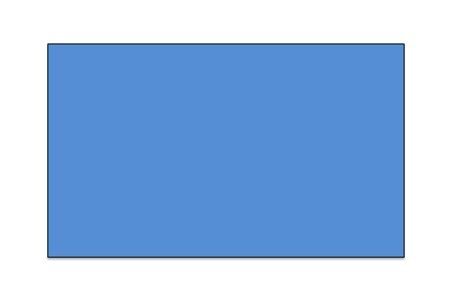## Rectangle### Description

The term rectangle normally refers to a quadrilateral with four right angles. This is a simple rectangle. Every rectangle has their opposite sides parallel and are equal in length, the two diagonals bisect each other and are equal in length. All angles are 90 degrees. Its area is its length multiplied by its breadth.

### References

Rectangle - For More Details